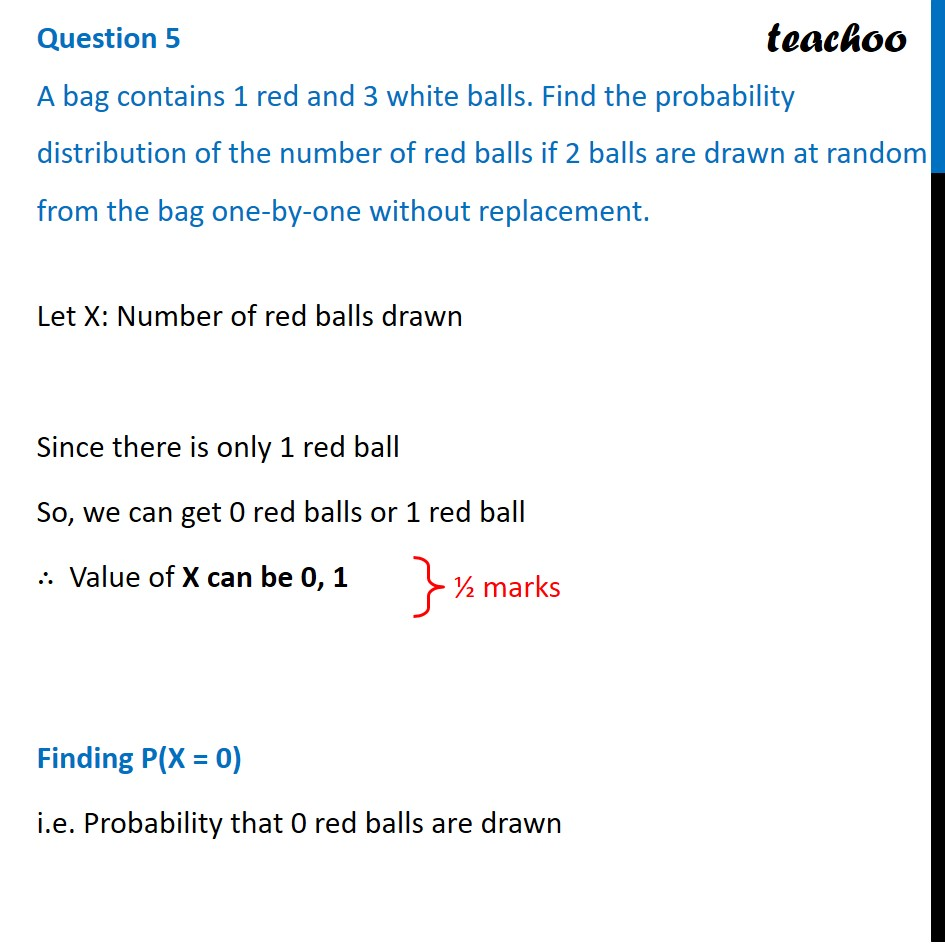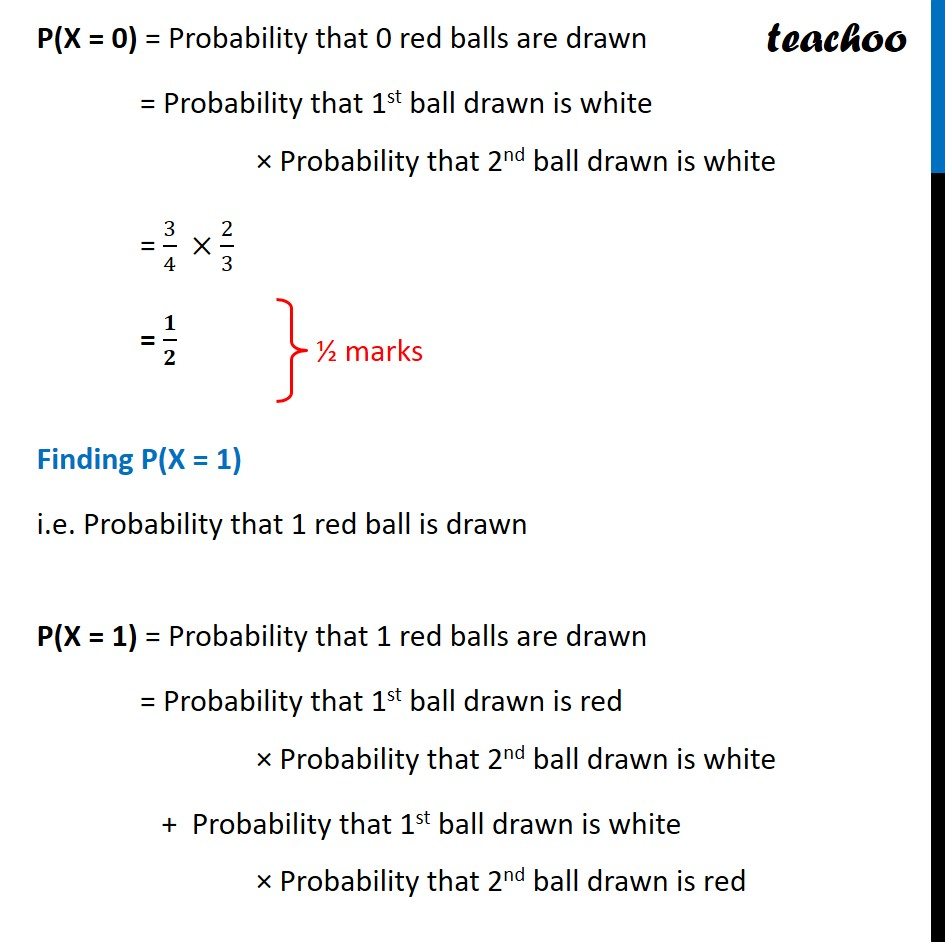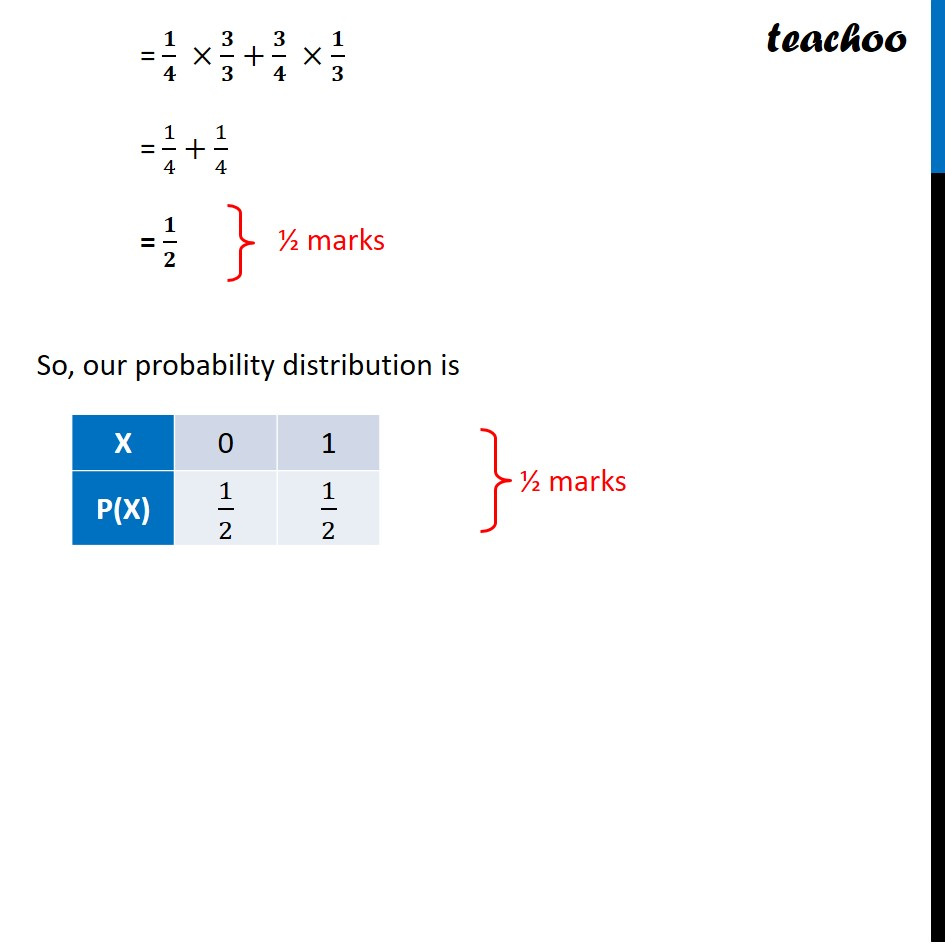CBSE Class 12 Sample Paper for 2022 Boards (For Term 2)

Class 12
Solutions of Sample Papers and Past Year Papers - for Class 12 Boards

## A bag contains 1 red and 3 white balls. Find the probability distribution of the number of red balls if 2 balls are drawn at random from the bag one-by-one without replacement.Get live Maths 1-on-1 Classs - Class 6 to 12

### Transcript

Question 5 A bag contains 1 red and 3 white balls. Find the probability distribution of the number of red balls if 2 balls are drawn at random from the bag one-by-one without replacement. Let X: Number of red balls drawn Since there is only 1 red ball So, we can get 0 red balls or 1 red ball ∴ Value of X can be 0, 1 Finding P(X = 0) i.e. Probability that 0 red balls are drawn ½ marks P(X = 0) = Probability that 0 red balls are drawn = Probability that 1st ball drawn is white × Probability that 2nd ball drawn is white = 3/4 ×2/3 = 𝟏/𝟐 Finding P(X = 1) i.e. Probability that 1 red ball is drawn P(X = 1) = Probability that 1 red balls are drawn = Probability that 1st ball drawn is red × Probability that 2nd ball drawn is white + Probability that 1st ball drawn is white × Probability that 2nd ball drawn is red ½ marks = 𝟏/𝟒 ×𝟑/𝟑+𝟑/𝟒 ×𝟏/𝟑 = 1/4+1/4 = 𝟏/𝟐 So, our probability distribution is